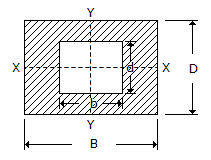Last updated: August 14, 2020

1. Coplaner concurrent forces are those forces which
2. When two elastic bodies collide with each other
3. The centre of gravity of a right angled triangle lies at its geometrical centre
4. The loss of kinetic energy due to direct impact of two bodies __________ upon the value of coefficient of restitution.
5. The force, by which the body is attracted, towards the centre of the earth, is called
6. The moment of inertia of a sphere of mass m and radius r, about an axis tangential to it, is
7. A machine which is capable of doing work in the reversed direction, after the effort is removed, is called a non-reversible machine.
8. The forces which do not meet at one point and their lines of action do not lie on the same plane are known as
9. Vectors method for the resultant force is also called polygon law of forces.
10. The power developed by a body acted upon by a torque T newton metre (N - m) and revolving at ω radian/s is given by
11. The time taken by a particle for one complete oscillation is known as periodic time.
12. If a pendulum is taken 1 km below the earth surface in a mine, it will __________ in time.
13. Mass moment of inertia of a thin rod about its one end is __________ the mass moment of inertia of the same rod about its mid-point
14. When a person, on a bicycle, drives round a curve, he has to lean __________ to maintain equilibrium.
15. The unit of power in S.I. units is
16. According to parallel axis theorem, the moment of inertia of a section about an axis parallel to the axis through centre of gravity (i.e. IP) is given by(where, A = Area of the section, IG = Moment of inertia of the section about an axis passing through its C.G., and h = Distance between C.G. and the parallel axis.)
17. The velocity ratio of a first system of pulleys with 4 pulleys is
18. The moment of inertia of a thin disc of mass m and radius r, about an axis through its centre of gravity and perpendicular to the plane of the disc is
19. Moment of inertia of a hollow rectangular section as shown in the below figure about Y-Y axis, is same as that about X-X axis.20. Two equal and opposite parallel forces whose lines of action are different form a couple.
Engineering Mechanics MCQ 9
You got {{userScore}} out of {{maxScore}} correct
{{title}}
{{image}}
{{content}}